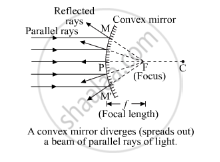Share

# Describe with a Suitable Diagram, How a Convex Mirror Diverges a Parallel Beam of Light Rays. Mark Clearly the Pole, Focus and Centre of Curvature of Concave Mirror in this Diagram. - CBSE Class 10 - Science

ConceptReflection of Light Image Formation by Spherical Mirrors - Convex Mirror

#### Question

Describe with a suitable diagram, how a convex mirror diverges a parallel beam of light rays. Mark clearly the pole, focus and centre of curvature of concave mirror in this diagram.

#### Solution

When a parallel beam of light, also parallel to the principle axis, is incident on a convex mirror, it will reflect from the mirror, and the reflected rays will appear to come from a point which is called the focus (F) of the convex mirror. So, a convex mirror has a virtual focus.

Ray diagram- A convex mirror diverges a parallel beam of light rays.Is there an error in this question or solution?

#### Video TutorialsVIEW ALL 

Solution Describe with a Suitable Diagram, How a Convex Mirror Diverges a Parallel Beam of Light Rays. Mark Clearly the Pole, Focus and Centre of Curvature of Concave Mirror in this Diagram. Concept: Reflection of Light - Image Formation by Spherical Mirrors - Convex Mirror.
S